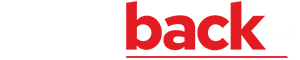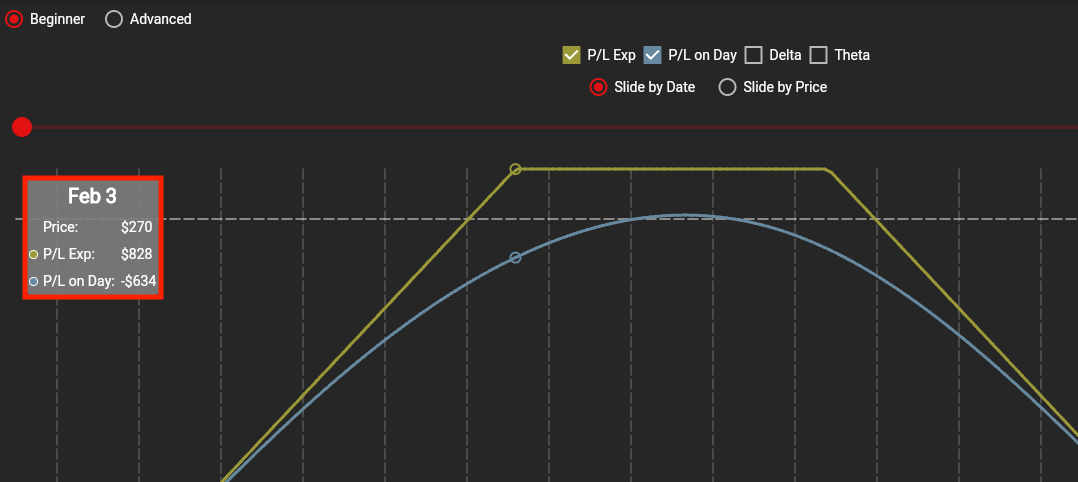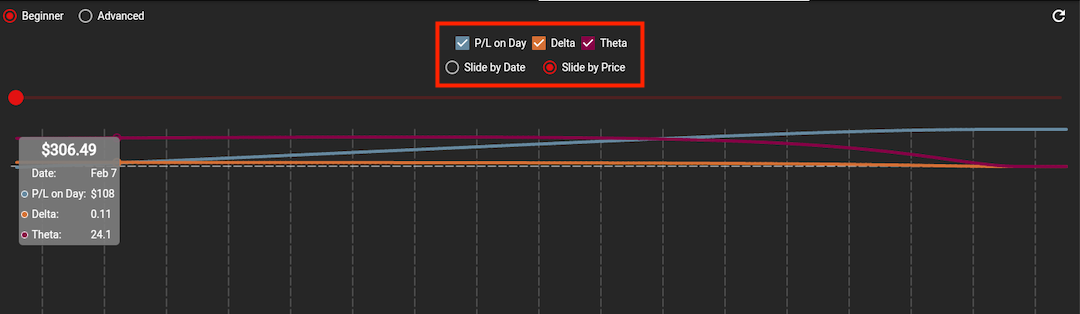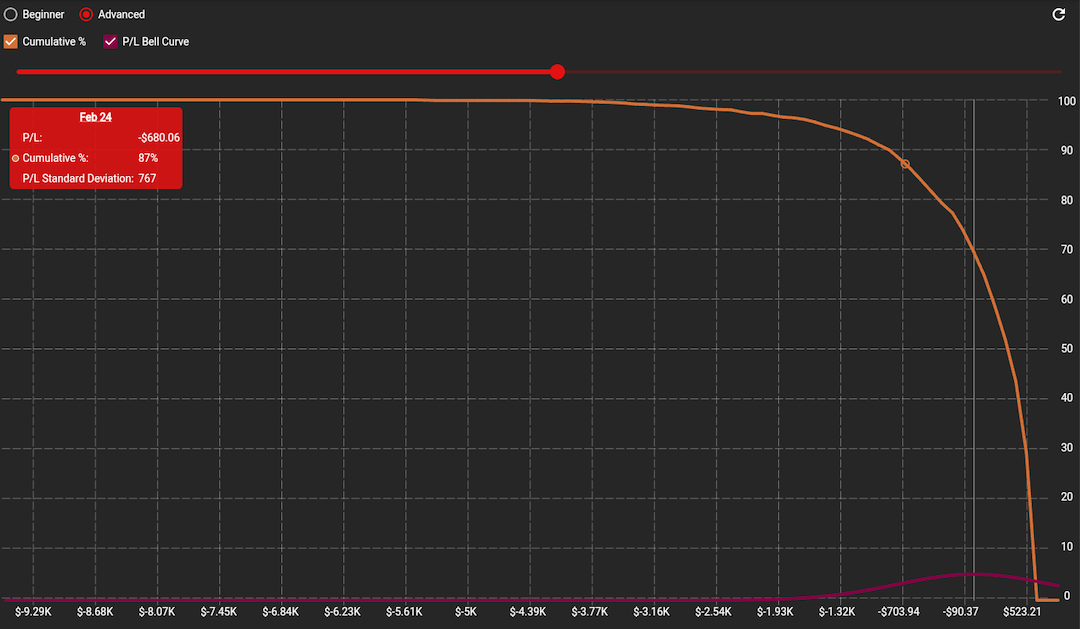# Analysis & Statistics

Analyze current positions to understand how price and time impacts your P/L.

## What is the Analysis & Statistics feature?

The analysis feature on lookback generates an interactive hockey stick graph that lets you show how changes in time or price impact your P/L, delta, and theta values. The advanced interface provides insight into the probability of making a profit by visualizing the expected standard deviation of your potential profit and loss between now and expiration.

• Analyze current positions and develop a stronger understanding of how price and time impacts their P/L.
• Adjust trade variables to see how their probability of profit changes, and how the greek values of delta and theta are influenced by changes.

## How to use the Analysis & Statistics feature?

#### 1. Utilize Analysis Tab

To utilize the “Analysis” tab, you’ll first have to enter a symbol in the top bar. Upon entering the symbol, in the “Chain” tab you can then choose a position that you would like to further analyze. Once you have the position selected, navigate to the “Analysis” tab. Here, you will see a theoretical profit and loss graph that shows the P/L at expiration and P/L on the day for the position you selected.#### 2. Adjust Slider for Date/Time/Price

The red slider above the graph allows you to adjust the date so that you can see how the P/L on the day changes with time. If you would like to see how delta and theta change with time, check the boxes at the top and you will see both of those lines appear on the graph. To see how delta and theta change with price, switch the slider to “Slide by Price” and you can see the impact that price has on the values of delta, theta, and your P/L on the day.#### 3. Gage Amount of Profit

Next, you can look at how likely it is that your position will make a certain amount of profit. Click on “Advanced” at the top of the page and you will see two lines, one representing cumulative percent and the other representing the P/L bell curve. The cumulative percent line shows the likelihood of you receiving a certain amount of profit/loss or greater, with the percent being on the y-axis. The x-axis represents the P/L, and the P/L bell curve line shows the distribution of profit/loss. The red slider above the graph allows you to slide by date, so you can see how the P/L bell curve flattens out and the percent chance of making a profit increases. We can use this graph to see how likely it is to make a certain profit by sliding to a specific date, and then locating the profit we would like to make.# TAKE THE GUESS OUT OF THE GUESSWORK

Start backtesting your strategies on Lookback today!